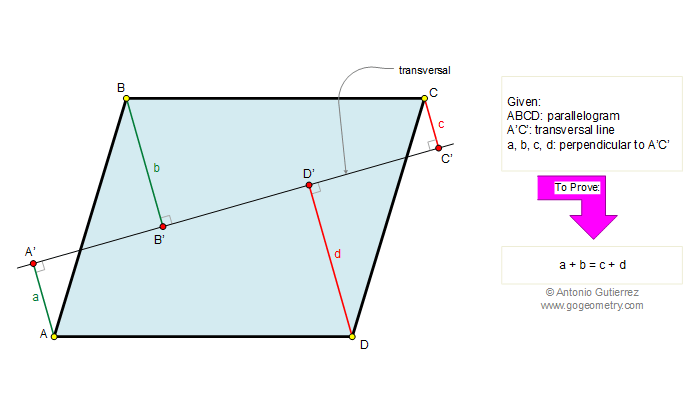Problem 235. Parallelogram, Transversal line, Perpendicular lines, Measurement The figure shows a parallelogram ABCD, A'C' is a transversal line. If a, b, c, and d are the perpendiculars drawn from A, B, C, and D to the line A'C', respectively, prove that: a + b = c + d. View or post a solution.Home | Geometry | Problems | 231-240 | Parallelogram | Email | by Antonio Gutierrez